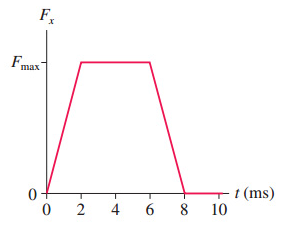# Problem: In (Figure 1), what value of Fmax gives an impulse of 3.0 N·s?Express your answer to two significant figures and include the appropriate units.

###### FREE Expert Solution

Impulse is equal to area under the curve:

There are three shapes under the curve: Triangle, rectangle and triangle:

I = (1/2)Fmax•Δt1 +Fmax•Δt2 + (`1/2)Fmax•Δt3

83% (106 ratings)###### Problem Details

In (Figure 1), what value of Fmax gives an impulse of 3.0 N·s?

Express your answer to two significant figures and include the appropriate units.